Drop a Query

# Linear Regression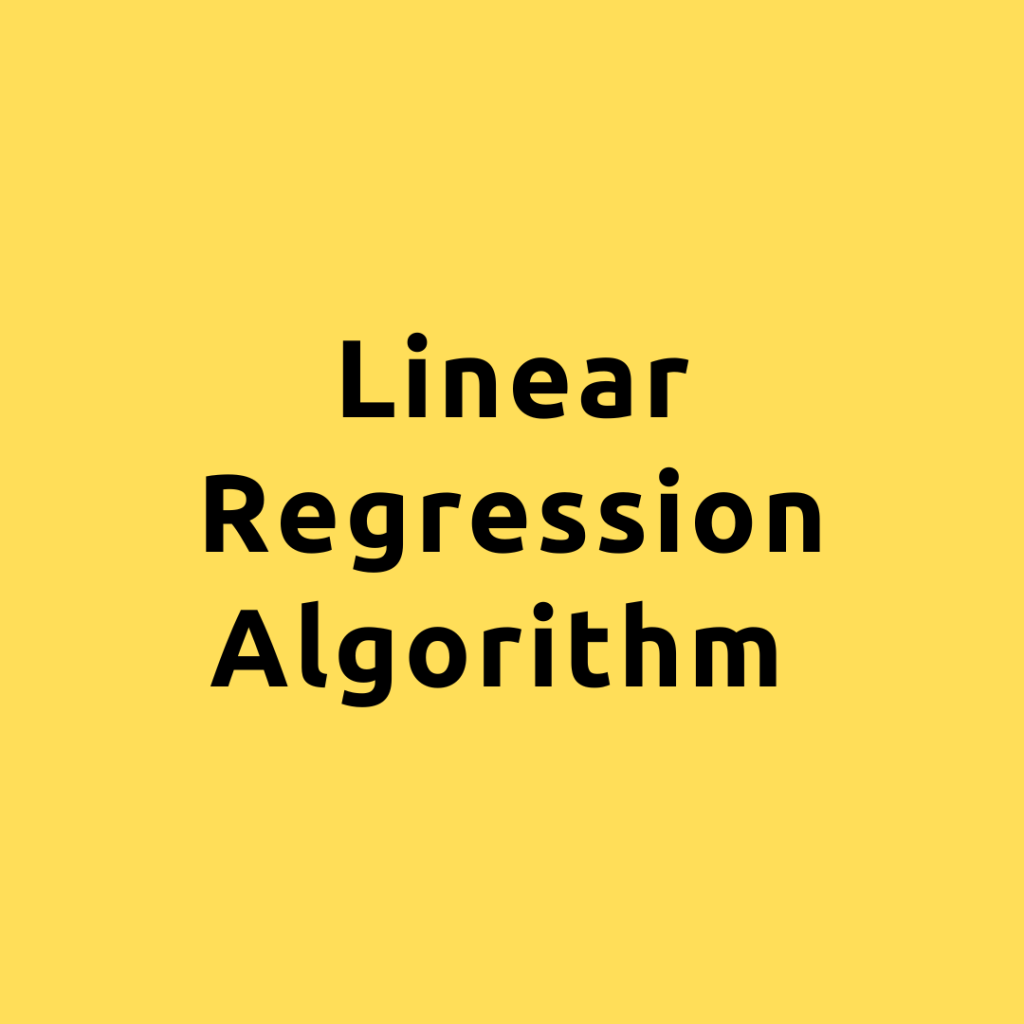## Linear Regression Algorithm – Applications and Concepts of Linear Algebra Using the Linear Regression Algorithm

Linear algebra, a branch of mathematics dealing with vectors and the rules for their operations, has many applications in the real world. One such application is in the field of machine learning, particularly in linear regression, a statistical method used to model the relationship between a dependent variable and one or more independent variables. In …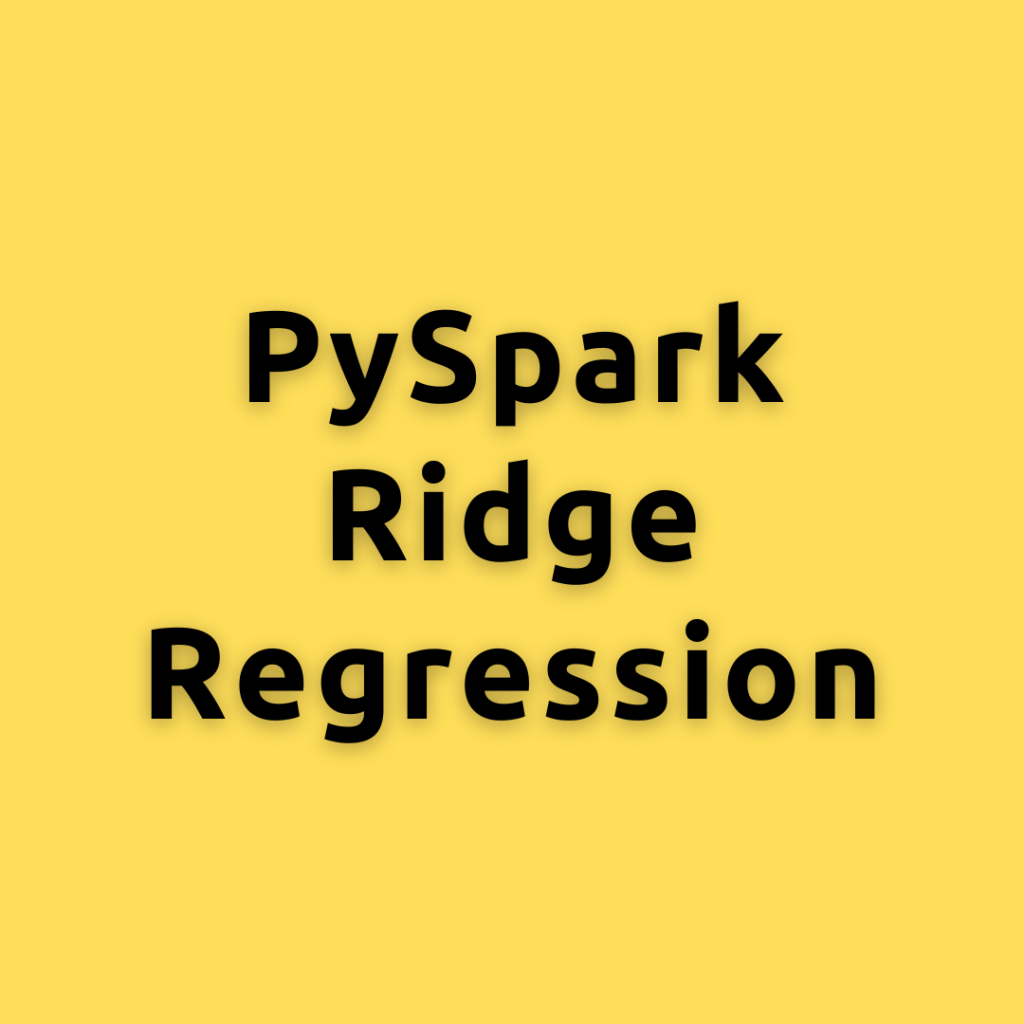## PySpark Ridge Regression – Building, Tuning, and Evaluating Ridge Regression with PySpark MLlib

Lets explore how to build, tune, and evaluate a Ridge Regression model using PySpark MLlib, a powerful library for machine learning and data processing in Apache Spark. Ridge Regression is an extension of linear regression that includes a regularization term to minimize the magnitude of the model’s coefficients and prevent overfitting. We will cover the …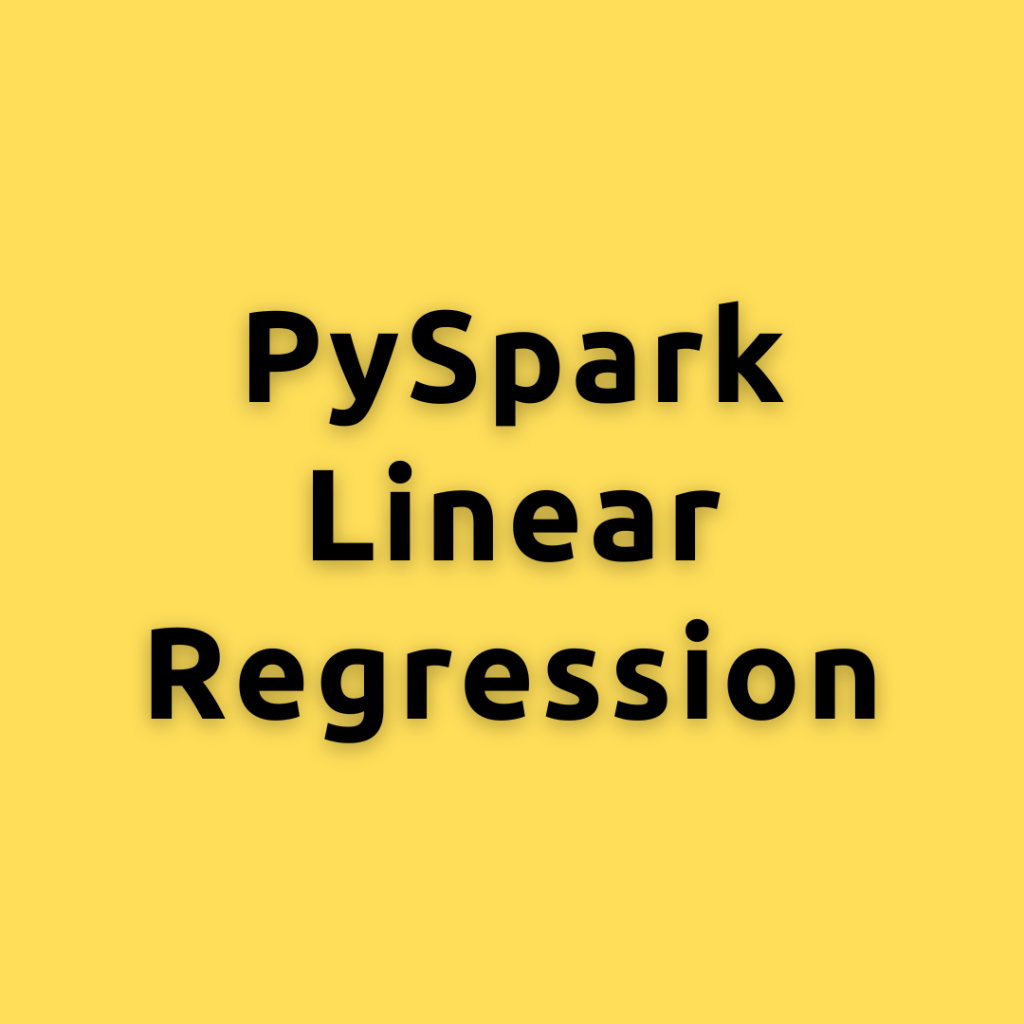## PySpark Linear Regression – How to Build and Evaluate Linear Regression Models using PySpark MLlib

MLlib, the machine learning library within PySpark, offers various tools and functions for machine learning algorithms, including linear regression. In this blog post, you will learn how to building and evaluating a linear regression model using PySpark MLlib with example code. Linear regression is a simple yet powerful machine learning algorithm used to predict a …

## Regression Coefficient Formula

Let’s understand the formula for the linear regression coefficients. That is the formula for both alpha and the beta. Now, if you have simple linear regression that does, you have just 1x variable in your data, you will be able to compute the values of alpha and beta using this formula. Let’s suppose you …

## Linear Regression in Machine Learning – Clearly Explained

In this lesson, I introduce what Linear regression is all about. Linear Regression is a foundational algorithm for machine learning and statistical modeling. Traditionally, Linear Regression is the very first algorithm you’d learn when getting started with predictive modeling. While there are a lot more ML and Deep learning algorithm in use today, …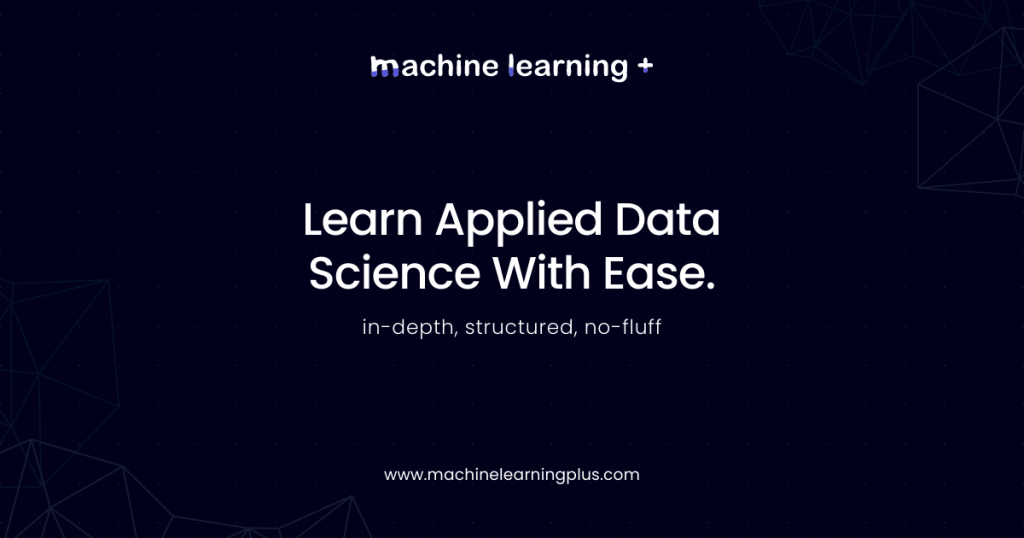## Linear Regression in Machine Learning – Clearly Explained

Understanding linear regression. Let’s understand what linear regression is all about from a non-technical perspective, before we get into the details, we will first understand from a layman’s terms what linear regression is. Now, linear regression is a machine learning algorithm ml algorithm that uses data to predict a quantity of interest, typically, we call …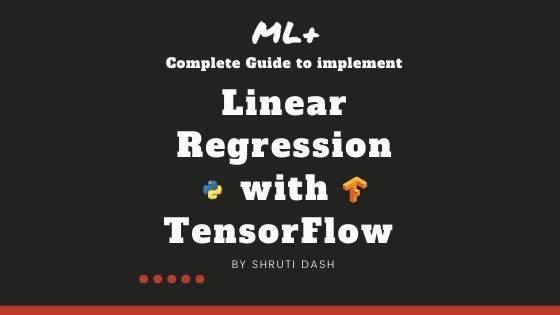## How to implement Linear Regression in TensorFlow

Linear Regression is one of the fundamental machine learning algorithms used to predict a continuous variable using one or more explanatory variables (features). In this tutorial, you will learn how to implement a simple linear regression in Tensorflow 2.0 using the Gradient Tape API. Overview In this tutorial, you will understand: Fundamentals of Linear Regression …## Linear Regression in Julia

Linear Regression is a fundamental machine learning algorithm used to predict a numeric dependent variable based on one or more independent variables. The dependent variable (Y) should be continuous. In this tutorial I explain how to build linear regression in Julia, with full-fledged post model-building diagnostics. To know more about the concepts behind linear regression, …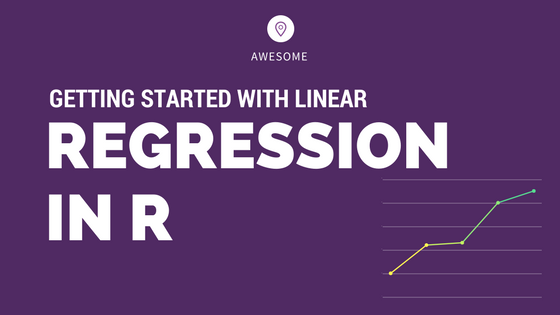## Complete Introduction to Linear Regression in R

We have covered the basic concepts about linear regression. Besides these, you need to understand that linear regression is based on certain underlying assumptions that must be taken care especially when working with multiple Xs. Once you are familiar with that, the advanced regression models will show you around the various special cases where a …Course Preview

## Machine Learning A-Z™: Hands-On Python & R In Data Science

### Free Sample Videos:#### Machine Learning A-Z™: Hands-On Python & R In Data Science#### Machine Learning A-Z™: Hands-On Python & R In Data Science#### Machine Learning A-Z™: Hands-On Python & R In Data Science#### Machine Learning A-Z™: Hands-On Python & R In Data Science